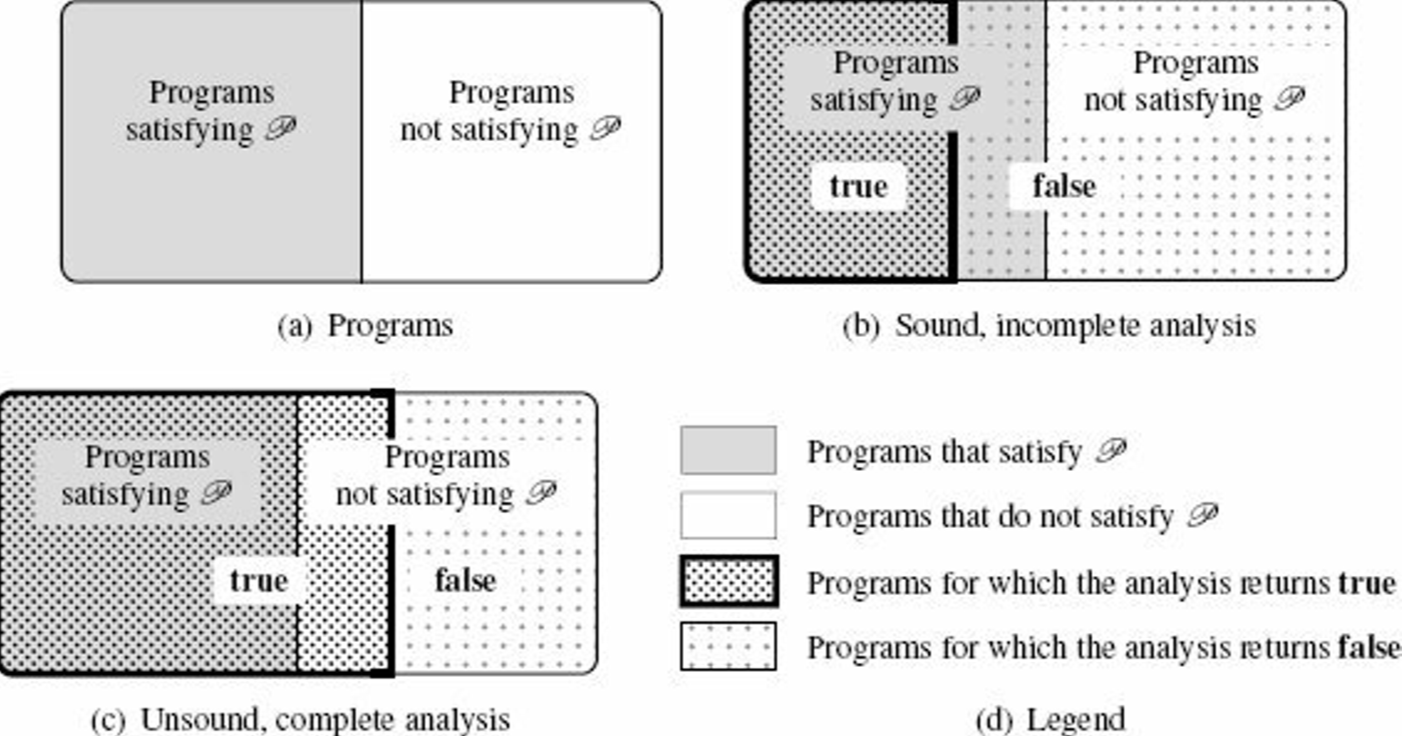\$ Chapter 1 — Program AnalysisChapter 1 — Program Analysis
17 April 2021

# 1.3 Concepts in Program Analysis

## 1.3.2 Static versus Dynamic

Motivations for static analysis

• "before-use" analysis

• analysis of a program that might be never terminating

## 1.3.3 A Hard Limit: Uncomputability

Theorem: Halting problem
The halting problem consists in finding an algorithm `halt` such that,
for every program `p` ∈ 𝙻, `halt`(`p`) = true if and only if `p` terminates

Theorem: Rice theorem
Let 𝙻 be a Turing-complete language, and let 𝒫 be a nontrivial semantic property of programs of 𝙻. There exists no algorithm such that,
for every program `p` ∈ 𝙻, it returns true if and only if `p` satisfies the semantic property 𝒫.

So how to design a program analysis ?

• target a restricted class of programs: give up the "for every program `p`" part

• not always being able to provide an exact answer: give up the "if and only if" part

## 1.3.5 Approximation: Soundness and Completeness

Let `analysis` to be an analysis tool to determine whether this property holds:

for every program `p` ∈ 𝙻, `analysis(p)` = true`p` satisfies 𝒫.
can be decomposed into a pair of implications:
for every program `p` ∈ 𝙻, `analysis(p)` = true`p` satisfies 𝒫.
for every program `p` ∈ 𝙻, `analysis(p)` = true`p` satisfies 𝒫.

## Soundness

A sound program analysis satisfies the first implication.

Definition: Soundness
The program analyzer `analysis` is sound with respect to property 𝒫 whenever, for any program `p` ∈ 𝙻, `analysis(p)` = true implies that `p` satisfies property 𝒫.

A sound analysis will reject all programs that do not satisfy 𝒫.

Example: Strong Typing
• good: well-typed programs will not present certain classes of errors

• bad: certain programs that will never crash may still be rejected

The soundness is easy to meet; we can simply reject any program. Therefore, in practice, the design of a sound analysis will try to give a conclusive answer as often as possible.

## Completeness

Definition: Completeness
The program analyzer `analysis` is complete with respect to property 𝒫 whenever, for every program `p` ∈ 𝙻, such that `p` satisfies 𝒫, `analysis(p)` = true.

The completeness is also easy to meet; we can simply never reject any program. To be useful, a complete analyzer should often reject programs that don't satisfy the property of interest.

## Soundness vs. CompletenessSoundness vs. Completeness (adapted from Figure 1.2 from the book)

When a program analysis is automatic, it is either unsound or incomplete.

# 1.4 Families of Program Analysis Techniques

• Testing: check a finite set of finite program executions

• unsound and complete

• good: easy, very close to the actual runtime

• bad: may not terminate, may not be deterministic (e.g. concurrent programs), not feasible to fully observe all executions

• xref: concolic testing to improve coverage and accuracy

• Assisted Proof: rely on user-supplied invariants

• 2 basic approaches:

• based on theorem-proving tools (e.g. Coq)

• leverages a tool infrastructure to prove a specific set of properties over programs in a specific language (e.g. dafny)

• good: often sound to respect to the model of the program semantics used for the proof, also complete up to the abilities of the proof assistant to verify proofs

• bad: non-automated, requires significant time and expertise

• (Finite-State) Model Checking: exhaustive exploration of finite systems

• use some kind of exhaustive (but efficient) enumeration and determine whether all executions satisfy the property of interest

• good: automatic, sound and complete with respect to the model

• caveat: verification is performed at the model level and not at the program level

• a model of the program needs to be constructed (manually or by some automatic frontend means)

• => the checking of the synthesized model may be either incomplete or unsound, with respect to the input program (incompleteness or unsoundness is often introduced in the modeling stage)

• often conservative: sound and incomplete with respect to the input program

• Conservative Static Analysis: automatic, sound, and incomplete approach

• idea: finitely over-approximate the set of all program behaviors using a specific set of properties

• many existing trials

• Astrée: proves the absence of run-time errors in embedded C codes

• Infer: detects memory issues in C/C++/Java programs

• JULIA: discovers security issues in Java programs

• often sound and incomplete

• we can think of unsound and complete static analysis

• will answer very different kind of question

• may guarantee that a given subset of the executions of the program can be observed, while it doesn't prove properties such as the absence of run-time errors

• bug finding: Relaxed error search, automatic, unsound, incomplete, based on heuristics

• simplify the design and implementation of analysis tools and to provide lighter-weight verification algorithms

• can be used to improve the quality of non-critical programs at a low cost

• examples:

• JET.jl: JET is unsound and incomplete bug finder for Julia, thus falls into this category (as of now, at least)

• CBMC: extracts models from C/C++/Java programs and performs bounded model checking (i.e. explores models only up to fixed depths)

automatic

soundness

completeness

object

when

testing

No

No

Yes

Program

Dynamic

Assisted Proof

No

Yes

Yes/No

Model

Static

Model Checking of finite-state model

Yes

Yes

Yes

Finite Model

Static

Model Checking at program level

Yes

Yes

No

Program

Static

Conservative Static Analysis

Yes

Yes

No

Program

Static

bug finding

Yes

No

No

Program

Static

an overview of program analysis techniques